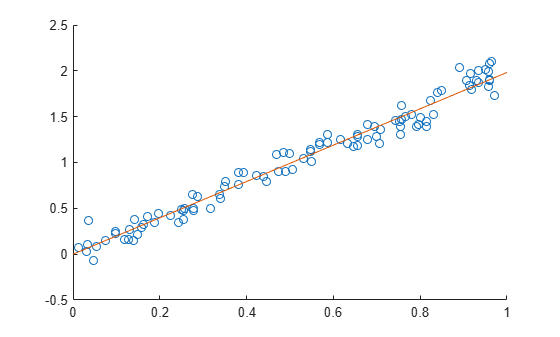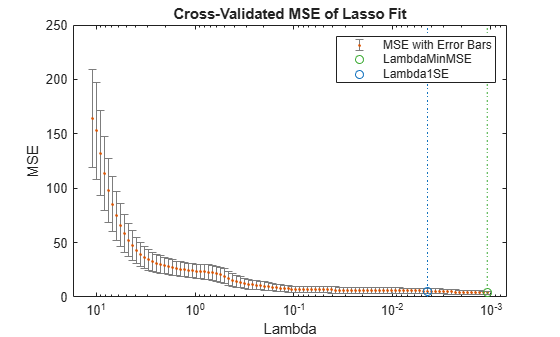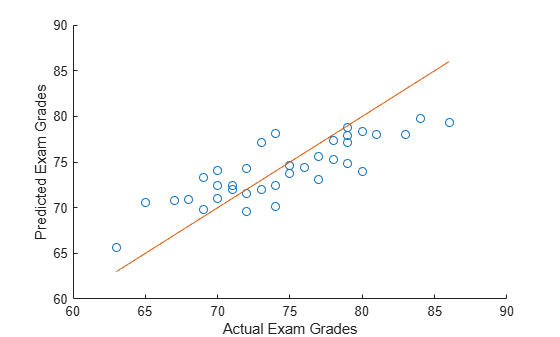# lasso

Lasso or elastic net regularization for linear models

## Syntax

``B = lasso(X,y)``
``B = lasso(X,y,Name,Value)``
``````[B,FitInfo] = lasso(___)``````

## Description

example

````B = lasso(X,y)` returns fitted least-squares regression coefficients for linear models of the predictor data `X` and the response `y`. Each column of `B` corresponds to a particular regularization coefficient in `Lambda`. By default, `lasso` performs lasso regularization using a geometric sequence of `Lambda` values.```

example

````B = lasso(X,y,Name,Value)` fits regularized regressions with additional options specified by one or more name-value pair arguments. For example, `'Alpha',0.5` sets elastic net as the regularization method, with the parameter `Alpha` equal to 0.5.```

example

``````[B,FitInfo] = lasso(___)``` also returns the structure `FitInfo`, which contains information about the fit of the models, using any of the input arguments in the previous syntaxes.```

## Examples

collapse all

Construct a data set with redundant predictors and identify those predictors by using `lasso`.

Create a matrix `X` of 100 five-dimensional normal variables. Create a response vector `y` from just two components of `X`, and add a small amount of noise.

```rng default % For reproducibility X = randn(100,5); weights = [0;2;0;-3;0]; % Only two nonzero coefficients y = X*weights + randn(100,1)*0.1; % Small added noise```

Construct the default lasso fit.

`B = lasso(X,y);`

Find the coefficient vector for the 25th `Lambda` value in `B`.

`B(:,25)`
```ans = 5×1 0 1.6093 0 -2.5865 0 ```

`lasso` identifies and removes the redundant predictors.

Create sample data with predictor variable `X` and response variable $y=0+2X+\epsilon$.

```rng('default') % For reproducibility X = rand(100,1); y = 2*X + randn(100,1)/10;```

Specify a regularization value, and find the coefficient of the regression model without an intercept term.

```lambda = 1e-03; B = lasso(X,y,'Lambda',lambda,'Intercept',false)```
```Warning: When the 'Intercept' value is false, the 'Standardize' value is set to false. ```
```B = 1.9825 ```

Plot the real values (points) against the predicted values (line).

```scatter(X,y) hold on x = 0:0.1:1; plot(x,x*B) hold off```Construct a data set with redundant predictors and identify those predictors by using cross-validated `lasso`.

Create a matrix `X` of 100 five-dimensional normal variables. Create a response vector `y` from two components of `X`, and add a small amount of noise.

```rng default % For reproducibility X = randn(100,5); weights = [0;2;0;-3;0]; % Only two nonzero coefficients y = X*weights + randn(100,1)*0.1; % Small added noise```

Construct the lasso fit by using 10-fold cross-validation with labeled predictor variables.

`[B,FitInfo] = lasso(X,y,'CV',10,'PredictorNames',{'x1','x2','x3','x4','x5'});`

Display the variables in the model that corresponds to the minimum cross-validated mean squared error (MSE).

```idxLambdaMinMSE = FitInfo.IndexMinMSE; minMSEModelPredictors = FitInfo.PredictorNames(B(:,idxLambdaMinMSE)~=0)```
```minMSEModelPredictors = 1x2 cell {'x2'} {'x4'} ```

Display the variables in the sparsest model within one standard error of the minimum MSE.

```idxLambda1SE = FitInfo.Index1SE; sparseModelPredictors = FitInfo.PredictorNames(B(:,idxLambda1SE)~=0)```
```sparseModelPredictors = 1x2 cell {'x2'} {'x4'} ```

In this example, `lasso` identifies the same predictors for the two models and removes the redundant predictors.

Visually examine the cross-validated error of various levels of regularization.

`load acetylene`

Create a design matrix with interactions and no constant term.

```X = [x1 x2 x3]; D = x2fx(X,'interaction'); D(:,1) = []; % No constant term```

Construct the lasso fit using 10-fold cross-validation. Include the `FitInfo` output so you can plot the result.

```rng default % For reproducibility [B,FitInfo] = lasso(D,y,'CV',10);```

Plot the cross-validated fits.

```lassoPlot(B,FitInfo,'PlotType','CV'); legend('show') % Show legend```The green circle and dotted line locate the `Lambda` with minimum cross-validation error. The blue circle and dotted line locate the point with minimum cross-validation error plus one standard deviation.

Predict students' exam scores using `lasso` and the elastic net method.

Load the `examgrades` data set.

```load examgrades X = grades(:,1:4); y = grades(:,5);```

Split the data into training and test sets.

```n = length(y); c = cvpartition(n,'HoldOut',0.3); idxTrain = training(c,1); idxTest = ~idxTrain; XTrain = X(idxTrain,:); yTrain = y(idxTrain); XTest = X(idxTest,:); yTest = y(idxTest);```

Find the coefficients of a regularized linear regression model using 10-fold cross-validation and the elastic net method with `Alpha` = 0.75. Use the largest `Lambda` value such that the mean squared error (MSE) is within one standard error of the minimum MSE.

```[B,FitInfo] = lasso(XTrain,yTrain,'Alpha',0.75,'CV',10); idxLambda1SE = FitInfo.Index1SE; coef = B(:,idxLambda1SE); coef0 = FitInfo.Intercept(idxLambda1SE);```

Predict exam scores for the test data. Compare the predicted values to the actual exam grades using a reference line.

```yhat = XTest*coef + coef0; hold on scatter(yTest,yhat) plot(yTest,yTest) xlabel('Actual Exam Grades') ylabel('Predicted Exam Grades') hold off```## Input Arguments

collapse all

Predictor data, specified as a numeric matrix. Each row represents one observation, and each column represents one predictor variable.

Data Types: `single` | `double`

Response data, specified as a numeric vector. `y` has length n, where n is the number of rows of `X`. The response `y(i)` corresponds to the ith row of `X`.

Data Types: `single` | `double`

### Name-Value Pair Arguments

Specify optional comma-separated pairs of `Name,Value` arguments. `Name` is the argument name and `Value` is the corresponding value. `Name` must appear inside quotes. You can specify several name and value pair arguments in any order as `Name1,Value1,...,NameN,ValueN`.

Example: `lasso(X,y,'Alpha',0.75,'CV',10)` performs elastic net regularization with 10-fold cross-validation. The `'Alpha',0.75` name-value pair argument sets the parameter used in the elastic net optimization.

Absolute error tolerance used to determine the convergence of the ADMM Algorithm, specified as the comma-separated pair consisting of `'AbsTol'` and a positive scalar. The algorithm converges when successive estimates of the coefficient vector differ by an amount less than `AbsTol`.

Note

This option applies only when you use `lasso` on tall arrays. See Extended Capabilities for more information.

Example: `'AbsTol',1e–3`

Data Types: `single` | `double`

Weight of lasso (L1) versus ridge (L2) optimization, specified as the comma-separated pair consisting of `'Alpha'` and a positive scalar value in the interval `(0,1]`. The value `Alpha = 1` represents lasso regression, `Alpha` close to `0` approaches ridge regression, and other values represent elastic net optimization. See Elastic Net.

Example: `'Alpha',0.5`

Data Types: `single` | `double`

Initial values for x-coefficients in ADMM Algorithm, specified as the comma-separated pair consisting of `'B0'` and a numeric vector.

Note

This option applies only when you use `lasso` on tall arrays. See Extended Capabilities for more information.

Data Types: `single` | `double`

Cross-validation specification for estimating the mean squared error (MSE), specified as the comma-separated pair consisting of `'CV'` and one of the following:

• `'resubstitution'``lasso` uses `X` and `y` to fit the model and to estimate the MSE without cross-validation.

• Positive scalar integer `K``lasso` uses `K`-fold cross-validation.

• `cvpartition` object `cvp``lasso` uses the cross-validation method expressed in `cvp`. You cannot use a `'leaveout'` partition with `lasso`.

Example: `'CV',3`

Maximum number of nonzero coefficients in the model, specified as the comma-separated pair consisting of `'DFmax'` and a positive integer scalar. `lasso` returns results only for `Lambda` values that satisfy this criterion.

Example: `'DFmax',5`

Data Types: `single` | `double`

Flag for fitting the model with the intercept term, specified as the comma-separated pair consisting of `'Intercept'` and either `true` or `false`. The default value is `true`, which indicates to include the intercept term in the model. If `Intercept` is `false`, then the returned intercept value is 0.

Example: `'Intercept',false`

Data Types: `logical`

Regularization coefficients, specified as the comma-separated pair consisting of `'Lambda'` and a vector of nonnegative values. See Lasso.

• If you do not supply `Lambda`, then `lasso` calculates the largest value of `Lambda` that gives a nonnull model. In this case, `LambdaRatio` gives the ratio of the smallest to the largest value of the sequence, and `NumLambda` gives the length of the vector.

• If you supply `Lambda`, then `lasso` ignores `LambdaRatio` and `NumLambda`.

• If `Standardize` is `true`, then `Lambda` is the set of values used to fit the models with the `X` data standardized to have zero mean and a variance of one.

The default is a geometric sequence of `NumLambda` values, with only the largest value able to produce `B` = `0`.

Example: `'Lambda',linspace(0,1)`

Data Types: `single` | `double`

Ratio of the smallest to the largest `Lambda` values when you do not supply `Lambda`, specified as the comma-separated pair consisting of `'LambdaRatio'` and a positive scalar.

If you set `LambdaRatio` = 0, then `lasso` generates a default sequence of `Lambda` values and replaces the smallest one with `0`.

Example: `'LambdaRatio',1e–2`

Data Types: `single` | `double`

Maximum number of iterations allowed, specified as the comma-separated pair consisting of `'MaxIter'` and a positive integer scalar.

If the algorithm executes `MaxIter` iterations before reaching the convergence tolerance `RelTol`, then the function stops iterating and returns a warning message.

The function can return more than one warning when `NumLambda` is greater than `1`.

Default values are `1e5` for standard data and `1e4` for tall arrays.

Example: `'MaxIter',1e3`

Data Types: `single` | `double`

Number of Monte Carlo repetitions for cross-validation, specified as the comma-separated pair consisting of `'MCReps'` and a positive integer scalar.

• If `CV` is `'resubstitution'` or a `cvpartition` of type `'resubstitution'`, then `MCReps` must be `1`.

• If `CV` is a `cvpartition` of type `'holdout'`, then `MCReps` must be greater than `1`.

Example: `'MCReps',5`

Data Types: `single` | `double`

Number of `Lambda` values `lasso` uses when you do not supply `Lambda`, specified as the comma-separated pair consisting of `'NumLambda'` and a positive integer scalar. `lasso` can return fewer than `NumLambda` fits if the residual error of the fits drops below a threshold fraction of the variance of `y`.

Example: `'NumLambda',50`

Data Types: `single` | `double`

Option to cross-validate in parallel and specify the random streams, specified as the comma-separated pair consisting of `'Options'` and a structure. This option requires Parallel Computing Toolbox™.

Create the `Options` structure with `statset`. The option fields are:

• `UseParallel` — Set to `true` to compute in parallel. The default is `false`.

• `UseSubstreams` — Set to `true` to compute in parallel in a reproducible fashion. For reproducibility, set `Streams` to a type allowing substreams: `'mlfg6331_64'` or `'mrg32k3a'`. The default is `false`.

• `Streams` — A `RandStream` object or cell array consisting of one such object. If you do not specify `Streams`, then `lasso` uses the default stream.

Example: `'Options',statset('UseParallel',true)`

Data Types: `struct`

Names of the predictor variables, in the order in which they appear in `X`, specified as the comma-separated pair consisting of `'PredictorNames'` and a string array or cell array of character vectors.

Example: `'PredictorNames',{'x1','x2','x3','x4'}`

Data Types: `string` | `cell`

Convergence threshold for the coordinate descent algorithm , specified as the comma-separated pair consisting of `'RelTol'` and a positive scalar. The algorithm terminates when successive estimates of the coefficient vector differ in the L2 norm by a relative amount less than `RelTol`.

Example: `'RelTol',5e–3`

Data Types: `single` | `double`

Augmented Lagrangian parameter ρ for the ADMM Algorithm, specified as the comma-separated pair consisting of `'Rho'` and a positive scalar. The default is automatic selection.

Note

This option applies only when you use `lasso` on tall arrays. See Extended Capabilities for more information.

Example: `'Rho',2`

Data Types: `single` | `double`

Flag for standardizing the predictor data `X` before fitting the models, specified as the comma-separated pair consisting of `'Standardize'` and either `true` or `false`. If `Standardize` is `true`, then the `X` data is scaled to have zero mean and a variance of one. `Standardize` affects whether the regularization is applied to the coefficients on the standardized scale or the original scale. The results are always presented on the original data scale.

If `Intercept` is `false`, then the software sets `Standardize` to `false`, regardless of the `Standardize` value you specify.

`X` and `y` are always centered when `Intercept` is `true`.

Example: `'Standardize',false`

Data Types: `logical`

Initial value of the scaled dual variable u in the ADMM Algorithm, specified as the comma-separated pair consisting of `'U0'` and a numeric vector.

Note

This option applies only when you use `lasso` on tall arrays. See Extended Capabilities for more information.

Data Types: `single` | `double`

Observation weights, specified as the comma-separated pair consisting of `'Weights'` and a nonnegative vector. `Weights` has length n, where n is the number of rows of `X`. The `lasso` function scales `Weights` to sum to `1`.

Data Types: `single` | `double`

## Output Arguments

collapse all

Fitted coefficients, returned as a numeric matrix. `B` is a p-by-L matrix, where p is the number of predictors (columns) in `X`, and L is the number of `Lambda` values. You can specify the number of `Lambda` values using the `NumLambda` name-value pair argument.

The coefficient corresponding to the intercept term is a field in `FitInfo`.

Data Types: `single` | `double`

Fit information of the linear models, returned as a structure with the fields described in this table.

Field in FitInfoDescription
`Intercept`Intercept term β0 for each linear model, a `1`-by-L vector
`Lambda`Lambda parameters in ascending order, a `1`-by-L vector
`Alpha`Value of the `Alpha` parameter, a scalar
`DF`Number of nonzero coefficients in `B` for each value of `Lambda`, a `1`-by-L vector
`MSE`Mean squared error (MSE), a `1`-by-L vector
`PredictorNames`Value of the `PredictorNames` parameter, stored as a cell array of character vectors

If you set the `CV` name-value pair argument to cross-validate, the `FitInfo` structure contains these additional fields.

Field in FitInfoDescription
`SE`Standard error of MSE for each `Lambda`, as calculated during cross-validation, a `1`-by-L vector
`LambdaMinMSE``Lambda` value with the minimum MSE, a scalar
`Lambda1SE`Largest `Lambda` value such that MSE is within one standard error of the minimum MSE, a scalar
`IndexMinMSE`Index of `Lambda` with the value `LambdaMinMSE`, a scalar
`Index1SE`Index of `Lambda` with the value `Lambda1SE`, a scalar

collapse all

### Lasso

For a given value of λ, a nonnegative parameter, `lasso` solves the problem

`$\underset{{\beta }_{0},\beta }{\mathrm{min}}\left(\frac{1}{2N}\sum _{i=1}^{N}{\left({y}_{i}-{\beta }_{0}-{x}_{i}^{T}\beta \right)}^{2}+\lambda \sum _{j=1}^{p}|{\beta }_{j}|\right).$`
• N is the number of observations.

• yi is the response at observation i.

• xi is data, a vector of length p at observation i.

• λ is a nonnegative regularization parameter corresponding to one value of `Lambda`.

• The parameters β0 and β are a scalar and a vector of length p, respectively.

As λ increases, the number of nonzero components of β decreases.

The lasso problem involves the L1 norm of β, as contrasted with the elastic net algorithm.

### Elastic Net

For α strictly between 0 and 1, and nonnegative λ, elastic net solves the problem

`$\underset{{\beta }_{0},\beta }{\mathrm{min}}\left(\frac{1}{2N}\sum _{i=1}^{N}{\left({y}_{i}-{\beta }_{0}-{x}_{i}^{T}\beta \right)}^{2}+\lambda {P}_{\alpha }\left(\beta \right)\right),$`

where

`${P}_{\alpha }\left(\beta \right)=\frac{\left(1-\alpha \right)}{2}{‖\beta ‖}_{2}^{2}+\alpha {‖\beta ‖}_{1}=\sum _{j=1}^{p}\left(\frac{\left(1-\alpha \right)}{2}{\beta }_{j}^{2}+\alpha |{\beta }_{j}|\right).$`

Elastic net is the same as lasso when α = 1. For other values of α, the penalty term Pα(β) interpolates between the L1 norm of β and the squared L2 norm of β. As α shrinks toward 0, elastic net approaches `ridge` regression.

## Algorithms

collapse all

When operating on tall arrays, `lasso` uses an algorithm based on the Alternating Direction Method of Multipliers (ADMM) . The notation used here is the same as in the reference paper. This method solves problems of the form

Minimize $l\left(x\right)+g\left(z\right)$

Subject to $Ax+Bz=c$

Using this notation, the lasso regression problem is

Minimize $l\left(x\right)+g\left(z\right)=\frac{1}{2}{‖Ax-b‖}_{2}^{2}+\lambda {‖z‖}_{1}$

Subject to $x-z=0$

Because the loss function $l\left(x\right)=\frac{1}{2}{‖Ax-b‖}_{2}^{2}$ is quadratic, the iterative updates performed by the algorithm amount to solving a linear system of equations with a single coefficient matrix but several right-hand sides. The updates performed by the algorithm during each iteration are

`$\begin{array}{l}{x}^{k+1}={\left({A}^{T}A+\rho I\right)}^{-1}\left({A}^{T}b+\rho \left({z}^{k}-{u}^{k}\right)\right)\\ {z}^{k+1}={S}_{\lambda /\rho }\left({x}^{k+1}+{u}^{k}\right)\\ {u}^{k+1}={u}^{k}+{x}^{k+1}-{z}^{k+1}\end{array}$`

A is the dataset (a tall array), x contains the coefficients, ρ is the penalty parameter (augmented Lagrangian parameter), b is the response (a tall array), and S is the soft thresholding operator.

`${S}_{\kappa }\left(a\right)=\left\{\begin{array}{c}\begin{array}{cc}a-\kappa ,\text{\hspace{0.17em}}& a>\kappa \end{array}\\ \begin{array}{cc}0,\text{\hspace{0.17em}}& |a|\text{\hspace{0.17em}}\le \kappa \text{\hspace{0.17em}}\end{array}\\ \begin{array}{cc}a+\kappa ,\text{\hspace{0.17em}}& a<\kappa \text{\hspace{0.17em}}\end{array}\end{array}.$`

`lasso` solves the linear system using Cholesky factorization because the coefficient matrix ${A}^{T}A+\rho I$ is symmetric and positive definite. Because $\rho$ does not change between iterations, the Cholesky factorization is cached between iterations.

Even though A and b are tall arrays, they appear only in the terms ${A}^{T}A$ and ${A}^{T}b$. The results of these two matrix multiplications are small enough to fit in memory, so they are precomputed and the iterative updates between iterations are performed entirely within memory.

 Tibshirani, R. “Regression Shrinkage and Selection via the Lasso.” Journal of the Royal Statistical Society. Series B, Vol. 58, No. 1, 1996, pp. 267–288.

 Zou, H., and T. Hastie. “Regularization and Variable Selection via the Elastic Net.” Journal of the Royal Statistical Society. Series B, Vol. 67, No. 2, 2005, pp. 301–320.

 Friedman, J., R. Tibshirani, and T. Hastie. “Regularization Paths for Generalized Linear Models via Coordinate Descent.” Journal of Statistical Software. Vol. 33, No. 1, 2010. `https://www.jstatsoft.org/v33/i01`

 Hastie, T., R. Tibshirani, and J. Friedman. The Elements of Statistical Learning. 2nd edition. New York: Springer, 2008.

 Boyd, S. “Distributed Optimization and Statistical Learning via the Alternating Direction Method of Multipliers.” Foundations and Trends in Machine Learning. Vol. 3, No. 1, 2010, pp. 1–122.